1.8 Review of Hypothesis Testing
1.8.1 Some Basics
We have the general linear model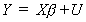1

where Y is nx1, X is nxk, b is kx1 and U is nx1. The population disturbance is distributed according to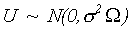So the Aitken estimator is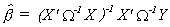which can be rewritten in terms of the population disturbance as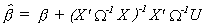(2)

The sampling distribution of our estimator, for suitable assumptions about the distribution of U or the sample size, is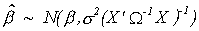We would estimate the scalar in the covariance matrix from either the least squares approach or the maximum likelihood approach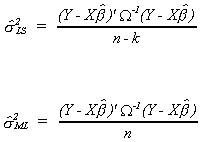Proceeding with the least squares estimator of the error variance, the quadratic form in the numerator can be written as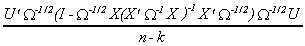or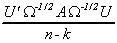8 (3)

which is distributed as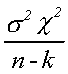with n-k degrees of freedom. Note that the matrix A has rank n-k.
Returning to (2), we can rearrange the expression for the estimator as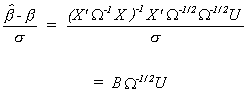which is distributed as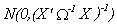You should be able to show with a bit of algebra that BA=0. This is, of course, the necessary and sufficient condition for a 2 and a normal to be independent. We also know that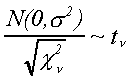1. The Garden Variety t-test
With these results in mind we can set up the general procedure for testing hypotheses about an arbitrary linear combination of the least squares coefficients.

Suppose we wish to test the following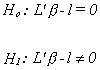where L is kx1 and l is a scalar. Our test statistic is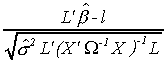where the estimate of the error variance is constructed from the residuals of the unrestricted regression.

The numerator is a linear combination of normal random variables and is therefore normally distributed. You may recall from equation (2) of the previous section that the estimator is a linear combination of random variables in the regression error vector. The same section shows that the denominator is a chi-square. Hence the ratio is a t-statistic.

1.8.3 The Garden Variety F-test

Suppose we have the null and alternate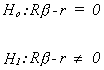where R is jxk and r is jx1. The rank of R must be j<k. That is, the joint set of restrictions must be linearly independent and there must be fewer of them than the number of unknown coefficients.
We construct an estimate of b for the unrestricted parameter space. I.e.,And we use this to construct an estimate of the restriction, i.e.,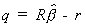Under the null we have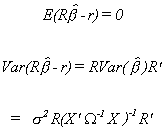We have been assuming normality so under null, in both small and large samples,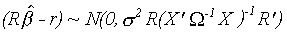Therefore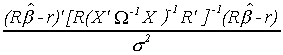is distributed as 2 with j degrees of freedom.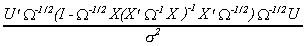to be 2 with n-k degrees of freedom so the ratio of the two will be F(j,n-k) with a noncentrality parameter of zero under the null hypothesis. We read the appropriate critical value from a central F table. Under the alternate hypothesis the test statistic is distributed non-centrally. This is a result of the fact that the numerator is constructed from squared normal random variables which do not have a zero mean. When the null is false, therefore, we get a draw from a distribution that is more highly skewed than the F under the null. That is, we are more likely to observe more extreme F-statistics. This is a small sample test.
It is also worth noting that the conventional F-test is very conservative. That is, the restrictions are believed to hold absolutely under the null. If they are incorrect by even a little bit then we want to reject the null. At the same time, we know that the variance of the restricted least squares estimator is smaller than that for the unrestricted OLS estimator. This suggests that the preferred estimator might not be that which has minimum variance among unbiased estimators and we might not want to base our test statistic on that estimator.

1. The Mean Square Error Test

By definition we have MSE = Variance + Bias2. Under the null hypothesis as usually stated, the restrictions are identically correct, we recognize that the MSE of the OLS estimator is the same as its variance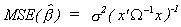For our restricted least squares estimator we had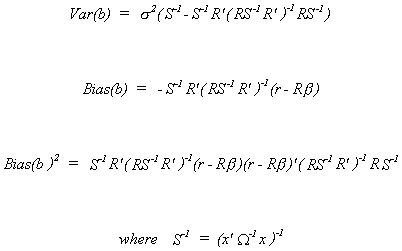Combining these we get the MSE for the RLS estimator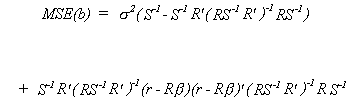We want to choose the estimator with the smaller MSE. Taking the difference between the two gives us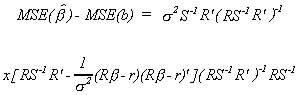25 (i)

So our null hypothesis is that the two MSE are the same and the alternate is that MSE for the RLS is greater than that for OLS.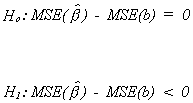Recalling (*), we see that it is the difference between two quadratic forms which can be factored as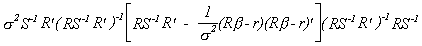We are interested in whether this is > 0. Think of it as a quadratic form of the sort B'AB, which we saw to be positive definite if, for B nonsingular, A was positive definite. So we are curious about the middle term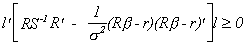for all l not identically zero. Or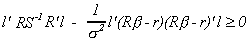Or,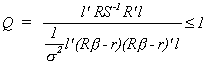From the Cauchy-Schwartz inequality we know that this ratio can never be greater than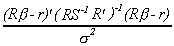31 (ii)

and reaches a maximum if we choose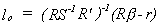If A is positive definite then we know Q 1 for any l, including lo. Since lo gives the maximum of Q and is known to be less than 1, we can write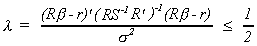Remember, in constructing the F-test we assumed that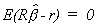. If it does not then the F-statistic is noncentral with noncentrality parameter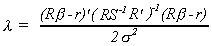This noncentrality parameter can be as big as �, on the basis of the argument above, before the difference between the MSEs goes negative. Thus we read our critical values from an F table with a noncentrality parameter equal to �.

1. The Minimum Risk Test

Our criterion for choice of estimator might be to compare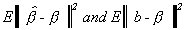These two terms are the average squared distance from our estimator to the true parameter value for OLS and RLS, respectively. They happen to be equal to the traces of the MSEs. So now we are interested in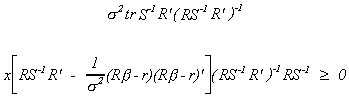we can show that this trace is positive only if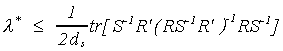38 (iii)

where ds is the smallest characteristic root of the matrix of which we are taking the trace. Our null is specified as (iii) being true. We construct the usual F statistic, but read our critical values from noncentral F tables with noncentrality parameters equal to the observed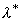.

1.8.6 Large Sample Tests

These lecture notes are only a primer. A very good survey article is Robert F. Engle, Chapter 13: Wald, Likelihood Ratio, and Lagrange Multiplier Tests in Econometrics, in Handbook of Econometrics, Vol. II, edited by Z. Griliches and M.D. Intriligator, Elsevier Publishers BV, 1984.

1.8.6.1 A Large Sample Estimator

Again we specifywhere Y is nx1, X is nxk, b is kx1 and U is nx1. The population disturbance is distributed according to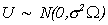The log likelihood for the sample is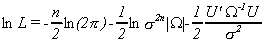We can do a change of variable and do some rearranging to get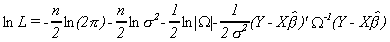Taking derivatives with respect to the unknown parameters gives us the well known first order conditions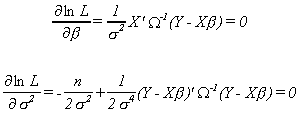Solving the first order conditions gives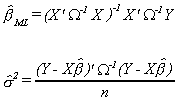Our parameter estimates then have the distribution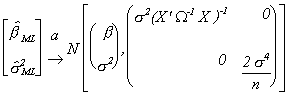1. Wald Test

We have either the maximum likelihood estimator or an OLS estimator and we believe Rb-r=0. An estimate of this happens to be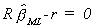which is distributed as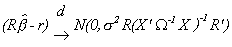under the null hypothesis.
So Wald suggests the test statisticThe estimate of the error variance is constructed using the residuals from the unrestricted model, i.e., the alternate hypothesis with all of the variables included. The reason is that you do no harm if you use the unrestricted model, even when the restrictions are true. Elsewhere we show that if you include irrelevant variables the least squares estimator remains BLU. However, if you omit relevant variables then your OLS estimator is biased. Then the linear combination of least squares estimates is not normal with mean zero, so the Wald statistic is not a central chi-square.

Through suitable manipulation we can write the Wald statistic aswhere e is the residual vector when we account for the restrictions under the null, U hat is the set of residuals when the parameter space is not restricted, and the estimate of sigma 2 is constructed from the residuals when the restrictions are not imposed.

1.8.6.3 The Likelihood Ratio Test

The likelihood function when there are no restrictions on the parameter space we know to be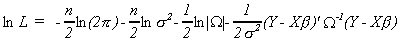In order to incorporate the restrictions in the likelihood function we could construct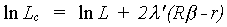where is a jx1 random vector. That is, there are j restrictions on the coefficient vector. Take partial derivatives with respect to the unknown parameters and set these first order conditions equal to zero. Solving the first order conditions we get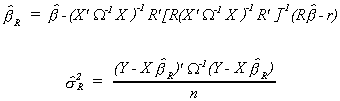so we are able to construct two estimates of the likelihood function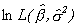from the unrestricted model andfrom the restricted model. The likelihood ratio statistic is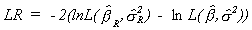With suitable manipulation we get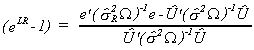Note that the estimate of the error variance is now based on both the residuals from the model with the restrictions under the null imposed and on the unrestricted model residuals. e and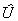have the previous definitions.

1.8.6.4 The Lagrange Multiplier Test
Returning to the Lagrangian of the previous section we have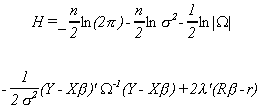The first order conditions from the maximization problem are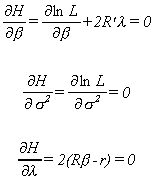The interpretation of l is that it is the gain in likelihood from easing the constraints. That is,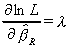Anyway, if we solve for l we get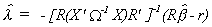which is distributed as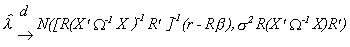Therefore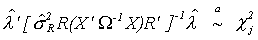The estimate of the error variance is based on the restricted model. Or, equivalently, is found from the first order conditions.
Suitable manipulation would give us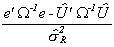where e andhave the previous definitions.

1.8.6.5 Relation Between the Tests

In the above example, if we were to know both W and s2, the three tests are numerically identical.

In the example as constructed, we had to estimate s2. The results show that although the three test statistics are c2 with j degrees of freedom, they will differ numerically in practice.

When both W and s2 are estimated then the differences between the observed values of the test statistics will differ more markedly.

There are potentially two sets of estimates of W. We can construct an estimate of W in the restricted parameter space, denote it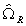. Or, it can be estimated in the unrestricted parameter space, denote it as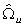. Then, in terms of residuals, the Wald statistic becomes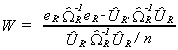where eR is the set of residuals when we usein the construction of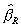and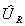is the set of residuals when we usein the construction of the unrestricted estimator, . The Lagrange multiplier statistic is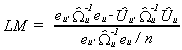where eu is the set of residuals when we usein the construction ofand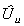is the set of residuals when we usein the construction of the unrestricted estimator,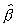. Finally, the likelihood ratio statistic is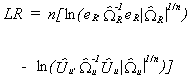Now it can be shown that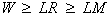but since they are all asymptotically c2 with the same degrees of freedom the critical point is always the same. Invariably this can lead to some conflict in whether one decides to reject the null.
There is also a graphical relationship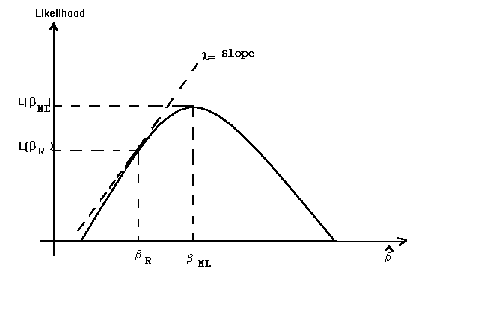The Lagrange multiplier is based on the slope of the likelihood function at the restricted estimator. The Likelihood ratio test is based on the difference of the likelihood function evaluated at the restricted and unrestricted estimates; i.e., the range of the likelihood. The Wald test is based on the difference between the restricted and unrestricted estimators; i.e. the domain of the likelihood function.

1.8.7 Decision Rules for Specification
Introduction
A truly classical statistician would be appalled by the use to which economists put Student's t and F tests. In the classical world the researcher formulates a hypothesis, collects data, estimates the model parameters, and tests the hypothesis. The presumption is that the hypothesis to be tested is a true reflection of the world. Regardless of the outcome of the test, the classical researcher does not use the test of hypothesis to respecify the model and reestimate from the same data.
In practice economists use the classical testing procedure as a decision rule for model selection: Choose that model which has the highest F; omit those variables which do not have significant t-statistics. The level of significance of the test, the probability of a Type I error is almost always somewhat arbitrarily chosen by the researcher. By choice of test and sample size the researcher is also choosing the probability of a Type II test. It is the choice of these two probabilities that determines the loss that the researcher is willing to incur in making an incorrect decision.
The situation may be pictured as in figure x.x. In the a (probability of a Type I error), b (probability of a Type II error) plane the convex line S represents the attainable error probabilities. The family of concave curves is a set of indifference curves showing the trade-off that the researcher is willing to make between the two types of errors. Utility increases as one moves closer to the origin. The optimal choice should be at E. If this should correspond to the usual 1%, 5% or 10% level of test chosen by researchers it would surely be coincidence.
If a model is to be chosen in a mechanistic fashion then the loss function should be clearly stated at the outset. One way to do this is to choose the model which is most informative about the data. An information criterion permits the researcher to choose a model under the double consideration of accuracy of estimation and best approximation to reality.

The Akaike Information Criterion (AIC)
Begin by defining g(Y) as the density function of the true probability distribution G(Y) for a vector random variable Y' = (y1, y2, ..., yn). Let f(Y|q) be a model for the unknown g(Y) where q e Q is a vector of parameters. Then a Kullback-Liebler Information Criterion (KLIC) for measuring the adequacy of the model is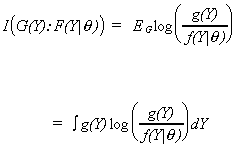The KLIC is a measure of the information received from an indirect message. That is, observing the proposed model tells us something about the unknown model. It is a decrasing function. Therefore, when the proposed model is close to the truth we are not surprised by the outcome and I(G(Y):F(Y|q)).
The obvious shortcoming is that the measure depends on the unknown g(Y). If the true density were known then it would be possible to establish a decision rule for choosing among alternative models. Suppose one is entertaining two possible models, f1(Y|q) and f2(Y|x). If I(G(Y): F1(Y|q)) < I(G(Y):F2(Y|x)) then the preferred model is f1(Y|q).
Because the KLIC depends on an unknown distribution function one must construct an estimator. Assume that I(G(Y): F(Y|q)) is twice continuously differentiable with respect to q. Define a pseudo true parameter as one that satifies the inequality I(G(Y): F(Y|qo)) < I(G(Y):F(Y|x)) for any qeQ. Satisfying this inequality is necessary and sufficient for qo to be pseudo true. The pseudo true parameters may be found from the evaluation of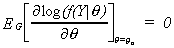.

Assuming that G(Y) = F(Y|q) almost everywhere, Akaike has shown that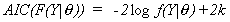is an almost unbiased estimator of the KLIC. k is the number of parameters in q. Given two alternative models one bases the decision on the model with the smallest Akaike Information Criterion.
Implied Critical Value of the AIC
Suppose that we are considering a general model, W, and its nested alternative, w, with k1 and k2 unknown coefficients, respectively. Our job is to choose the better of the two on the basis of the empirical results from a sample of size n. We can write the difference between the AICs for the two models as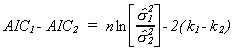When the difference is less than zero we choose W and when it is greater than zero we choose w. Making one's decision on this basis is equivalent to checking the inequality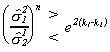Or, raising both sides to the n power and subtracting 1 from both sides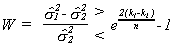If the random variable on the left is less than the right hand side then one chooses model W. Since the random variable W is based on sums of squared residuals it is known to have an F distribution, multiplied by a constant. In particular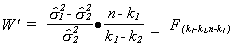Therefore the implied critical value in using the minimum AIC decision rule is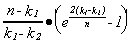One can compute this for any given problem and look up the probability in the appropriate table. For example, suppose k1=5, k2 = 3 and n=30. The implied critical value is 1.78 for an F with 2 and 25 degrees of freedom. This is an implied level of test of about 20%. This is a much higher level of test than would have been chosen by anyone applying the conventional F-test in a model selection application. Since the implied critical value is an increasing function of n it is obvious that with an increasing sample size the level of the test will decline.